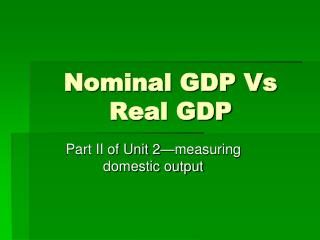DownloadDownload PresentationNominal GDP Vs Real GDP

# Nominal GDP Vs Real GDP

Télécharger la présentation## Nominal GDP Vs Real GDP

- - - - - - - - - - - - - - - - - - - - - - - - - - - E N D - - - - - - - - - - - - - - - - - - - - - - - - - - -
##### Presentation Transcript

1. Nominal GDP Vs Real GDP Part II of Unit 2—measuring domestic output

2. GDP • Reminder: GDP is a figure including every item produced in the economy. • Money is the common denominator that allows us to add the total output.

3. Nominal GDP • Is the market value of all final g & s produced in a year. • Calculated using current prices when the output was produced • Includes inflation • It is hard to compare market values from year to year when the value of the \$ itself changes (inflation or deflation) • To measure changes in the quantity of output, we need a “yardstick” that stays the same size.

4. Real GDP • The value of the final g & s produced in a given year expressed in the prices of a base year • 2000 for our example Nominal Vs Real

5. Traditional Method of Calculating GDPr • This economy produces apples & oranges • The base year is 2000. Since 2000 is the base year, real and nominal GDP are the same. • Only during the base year, will the GDPr and GDPn be the same

6. To find the GDPr in 2000, + the value of apples & oranges produced in 2000 using the table: Value of apples = 60 apples X \$.50 = \$30 Value of oranges = 80 oranges X \$.25 = \$20 • GDPr in 2000 = \$30 + \$20 = \$50

7. To calculate GDPr in 2006, + the value of apples and oranges using the prices of 2000 • Value of apples = 160 apples X \$.50 = \$80 • Value of oranges = 220 oranges X \$.25 = \$55 • GDPr in 2006 = \$80 + \$55 = \$135 • Nominal would be? • \$600

8. 2 purposes of estimating Real GDP • To compare the standard of living over time (based on quantity, not price) • To compare the standard of living among countries

9. Price Index • A measure of the price of a specified collection of g & s (market basket) in a given year as compared to the price of an identical collection of g & s in a reference year. • PI = price of market basket for a specific year X 100 price of same market basket in the base year • Find GDPr = GDPn / PI X 100

10. GDP Deflator • An average of current prices expressed as a percentage of base year prices. • Measures the price level • The average level of prices • GDP deflator = (GDPn / GDPr) X 100 • Example (\$100 / \$80) X 100 = GDP deflator • 1.25 X 100= 125 • Prices have gone up 25%

11. GDPr and the Price Level • Deflating the GDP Balloon • GDPn increases because of production, GDPr will increase.

12. GDPr and the Price Level • Deflating the GDP Balloon • GDPn also increases because prices rise.

13. GDPr and the Price Level • Deflating the GDP Balloon • We use the GDP deflator to let the air out of the nominal GDP balloon and reveal GDPr.

14. (Periods of falling real GDP) GDPr in the United States Billions of 1992 Dollars 8,000 7,000 Peak Trough 6,000 5,000 Recession Expansion 4,000 3,000 1970 1975 1980 1985 1990 1995 2000

15. The Consumer Price Index(CPI) • Index the gov’t uses to measure inflation • Gov’t uses it to adjust SS benefits and income tax brackets • Reports 300 items in a market basket • Index or base year always = 100

16. Inflation • A rise in the general level of prices • Inflation rate = current CPI-Index CPI = rate (X 100)= % index CPI or Year2 – Year1 = rate (X 100) = % Year1 orYearAfter – YearBefore= rate (X 100) = % YearBefore